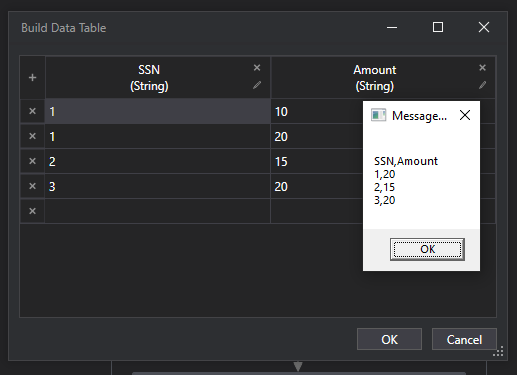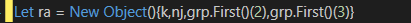# How to find the maximum value for an Unique ID using linq

I have a datatable mentioned as below

SSN Amount
1 10
1 20
2 10
3 9

I need to find the Max value of “Amount” column with respect to each distinct SSN and output the row to a datatable

expected output
SSN Amount
1 20
2 10
3 9

Hi @anjana.k ,

The case seems to be of Group By and Selecting the Max value out of the Grouped values.

For a Starter, Check the below links on Grouping :

@anjana.k
Welcome to Uipath community

Preparing of the Output datatable would be required, Here since the datatable schema is the same, we can take the clone of the Input datatable.

We can then use the below Expression :

``````(From d In dtData.AsEnumerable
Group d By k=d("SSN").toString.Trim Into grp=Group
Let value = grp.Max(Function (x) CDbl(x("Amount").toString))
Let ra = New Object(){k, value}
``````

Here, `dtData` is your Input datatable, and `dtData_New` is your Output datatable which should be the clone of the input datatable.

Hi,

FYI, another approach:

``````dt = dt.AsEnumerable.GroupBy(Function(r) r("SSN").ToString).Select(Function(g) g.OrderBy(Function(r) Int32.Parse(r("amount").ToString)).Last()).CopyToDataTable()
``````

Sample20221007-4.zip (2.8 KB)

Regards,

Check out the Workflow

HigestValue.xaml (7.6 KB)

OutputRegards
Gokul

Hi @Gokul001 ,
I have n number of columns in my real table, and i want all the columns to be present in the output table too

You can try `grp.First()(Column Index)`Regards
Gokul

Have you tried out @Yoichi 's Workflow/Suggestion provided above ?

Let us know if you are facing any difficulties.

1 Like

Hi @Yoichi ,
When i tried giving decimal values , it is throwing an error , also if any empty cells are found then also the bot throws error

@anjana.k Please find the below code

DT.AsEnumerable.GroupBy(Function(r) r(“SSN”).ToString).Select(Function(g) g.OrderBy(Function(r) Double.Parse(“0”&r(“amount”).ToString)).Last()).CopyToDataTable()

1 Like

Hi,

``````dt = dt.AsEnumerable.GroupBy(Function(r) r("SSN").ToString).Select(Function(g) g.OrderBy(Function(r) if(Double.TryParse(r("amount").ToString,New Double) ,Double.Parse(r("amount").ToString),Double.MinValue)).Last()).CopyToDataTable()